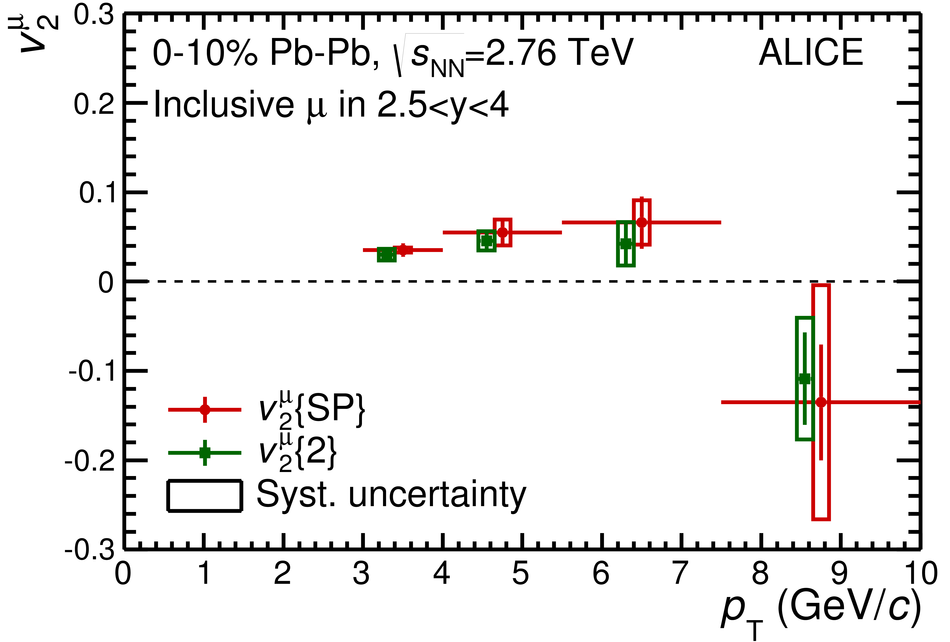# Figure 1

 $p_{\rm T}$-differential inclusive muon $v_2$ in $2.5 < y < 4$ and various centrality intervals, in Pb-Pb collisions at $\sqrt {s_{\rm NN}}$ = 2.76 TeV. The symbols are placed at the centre of the $p_{\rm T}$ interval and, for visibility, the points from two-particle $Q$ cumulants and Lee-Yang zeros with product generating function are shifted horizontally. The vertical error bars represent the statistical uncertainty, the horizontal error bars correspond to the width of the bin (not shown for the shifted data points) and the open boxes are the systematic uncertainties The $p_{\rm T}$ intervals used with the Lee-Yang zeros method are different with respect to the other methods Upper panels: results from two-particle correlation flow methods (scalar product and two-particle $Q$ cumulants)in the 0-10% (left) and 10-20% (right) centrality intervals Lower panels: results in the 20-40% centrality interval from two-particle correlation flow methods (scalar product and two-particle $Q$ cumulants) and from four-particle $Q$ cumulants (left), and from four-particle $Q$ cumulants and Lee-Yang zeros (right).